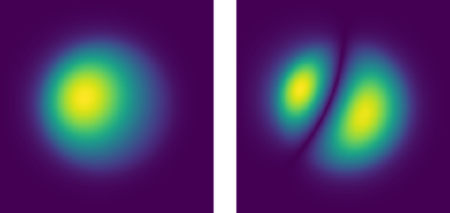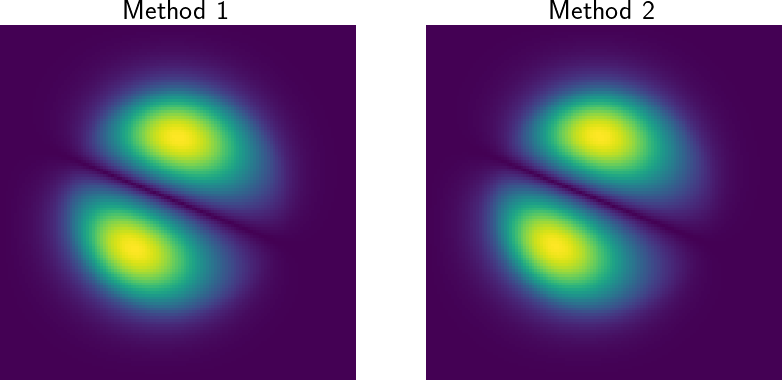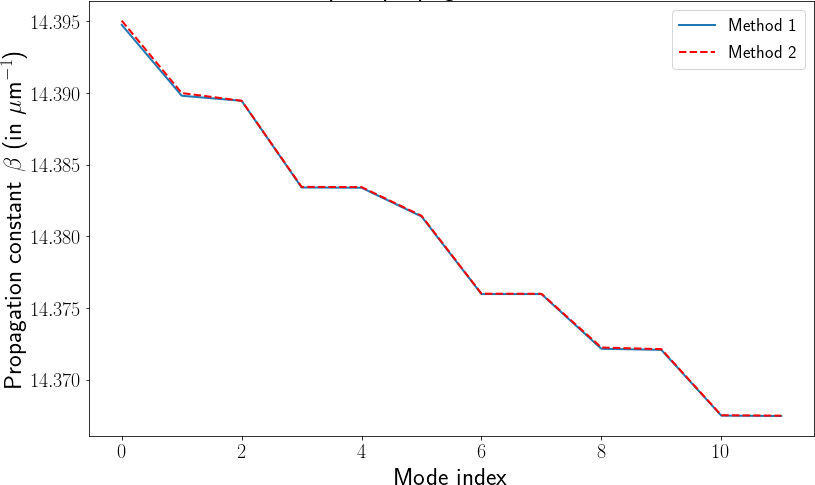## Compare Different Methods of Modes Estimation of Bent Multimode Fibers with pyMMFIn a previous tutorial, I explained how to calculate the modes of a bent multimode fibers. I introduced two methods, following the approach published in [M. Plöschner, T. Tyc, and T. Čižmár, Nat. Photon. (2015)]. In this short tutorial, I show how to use pyMMF to simulate bent fibers and compare the two different methods. A Jupyter notebook can be found on my Github account: compare_bending_methods.ipynb.

## Context

In the tutorial Numerical Estimation of Multimode Fiber Modes and Propagation Constants: Bent Fibers, I show that in order to find the propagation mode of a multimode fiber exhibiting bending we need to introduce the geometrical effect of bending as well as the compression effect. It can be done in two ways:

1. Method 1. Calculating the eigenmodes of the operator representing the Helmholtz equation in the presence of bending. As this operator is represented in the numerical code as a matrix of size the square of the number of discretization points, this computation can be quite time-consuming.

2. Method 2. If one already calculated the modes of the straight fiber, we can express the modified modes in the representation of the straight fiber modes and solve an eigenvalue problem in this lower dimension space. While it requires solving previously the problem for the straight configuration, it drastically reduces computation time when we want to calculate multiple bending configurations of the same fiber.

## Method 1

We solve the system with a given curvature state. Note that curvature can be set for both directions, e.g.  curvature = [2e4,5e4] (in microns).

modes_bent = solver.solve(nmodesMax=Nmodes,boundary = 'close',
mode = 'eig', curvature = curvature,
propag_only=False)
modes_bent.sort()
betas_bent = modes_bent.betas
profiles_bent = modes_bent.getModeMatrix()

## Method 2

While this method allows faster computation when trying to solve the same system for multiple configurations, it is important to keep in mind that

1. It uses more restrictive approximations, hence will give less accurate results, especially for lower curvatures,
2. It requires to find more than just the propagating modes of the straight system as it is not a full representation of the transverse space. This point is discussed in the tutorial.

First, we calculate the modes of the straight fiber:

modes_straight = solver.solve(nmodesMax=Nmodes_estim+20,boundary = 'close',
mode = 'eig', curvature = None,
propag_only=False)
Nmodes = sum(modes_straight.propag)
# Compute for the bent fiber the same number of modes as for the straight one
modes_straight.sort()

Note the propag_only=False that ensures that the solver returns all the modes calculated, i.e. the Nmodes_estim+20 modes that have the lowest real part of the propagation constant. This means that it returns also some non-propagating modes. These modes are sensitive to the boundary conditions of the system, hence they are a potential source of error.

We now find the new modes:

betas_bent2,profiles_bent2 = modes_straight.getCurvedModes(npola = 1,
curvature=curvature)

We finally only keep the propagating modes.

betas_bent2,profiles_bent2 = betas_bent2[:Nmodes],profiles_bent2[:,:Nmodes]

## Comparing the two methods

We compare here the results of both methods for the shape of the modes (Fig. 1) and the propagation constants (Fig. 2).Figure 1. Comparison of the second mode profile calculated using both methods.Figure 2. Comparison of the propagation constants calculated using both methods.

## Full code

The full code is available as a Jupyter notebook on my Github account: compare_bending_methods.ipynb

Created by sebastien.popoff on 26/05/2019